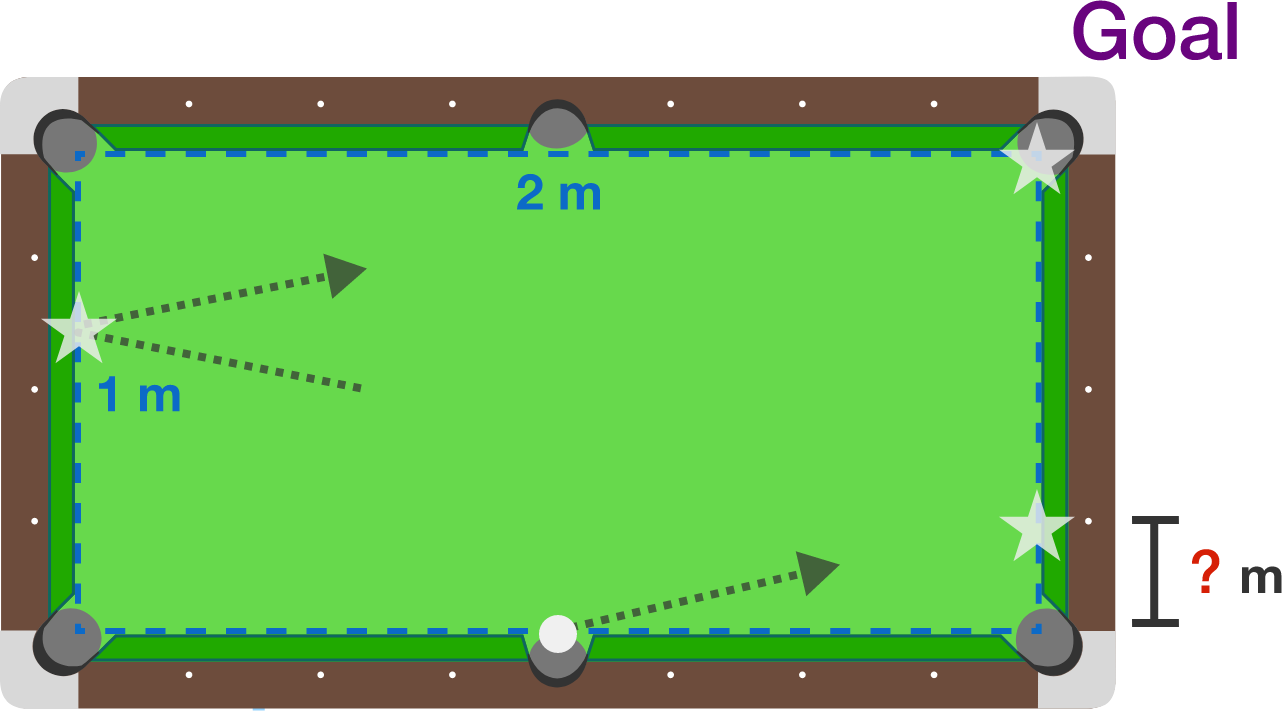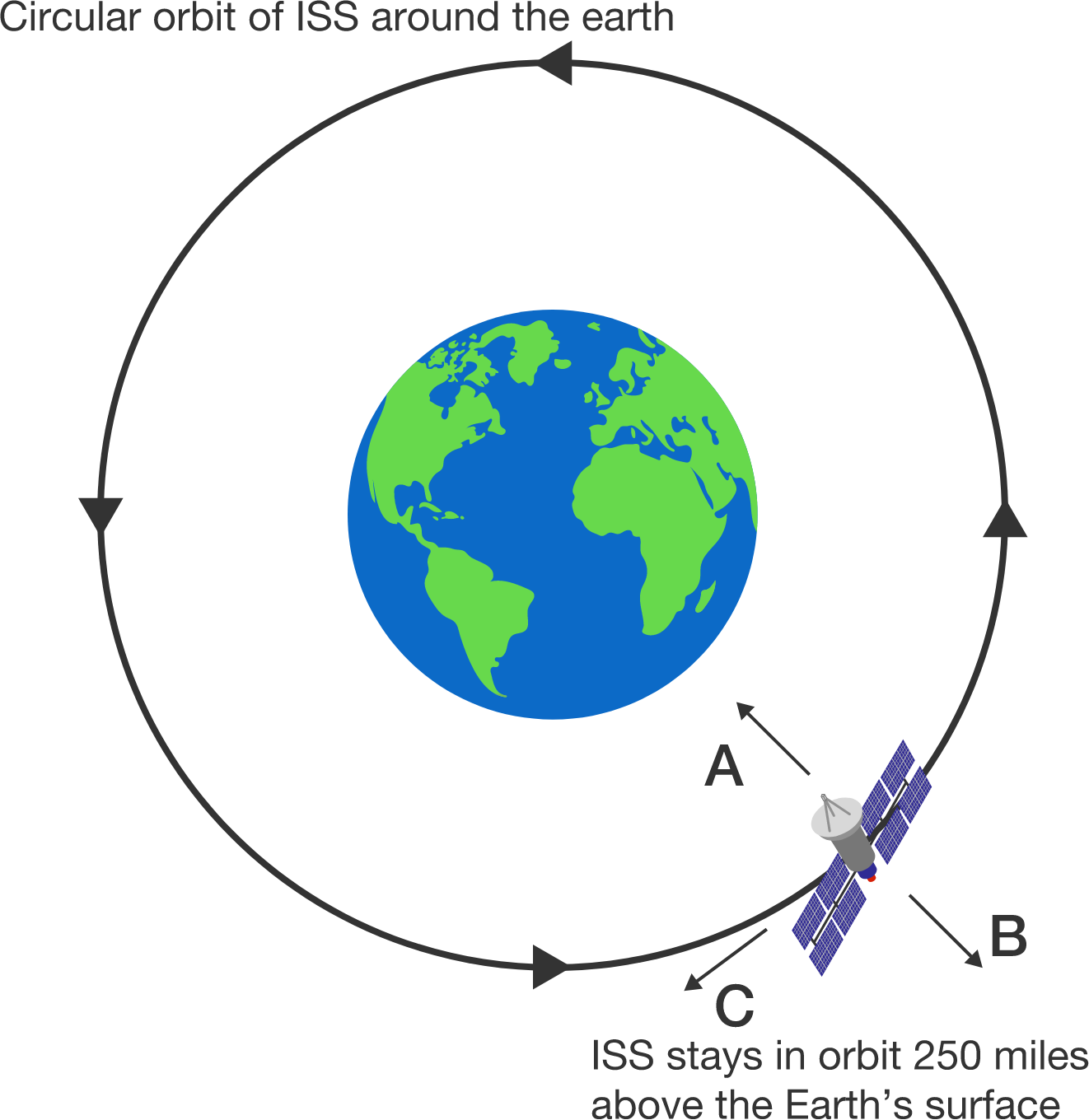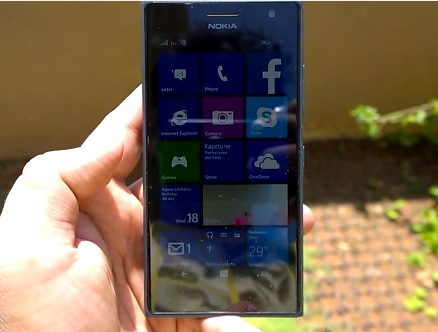# Problems of the Week

Contribute a problem

# 2018-01-15 BasicShelley has a ball placed at the exact bottom middle of a 2 m by 1 m pool table as shown. She wants to hit the ball towards the right side of the table such that it bounces exactly 2 times and ends up in the upper right hole. How far up from the lower right corner should she shoot the ball?

This problem is part of the Brilliant.org Open Problems Group. The end goal for each open problem is to find a solution, and maybe publish it if it's a nice enough result! You can read about the unsolved billiards problem that this one is related to here.

The International Space Station (ISS) orbits the earth in a circular orbit at uniform speed.

Suppose that an astronaut releases a ball from rest with respect to the center of the ISS. In what direction will the ball appear to move immediately after release, according to the astronaut?$1^2 + 2^2$ is not divisible by $1+2.$
$2^2 + 3^2$ is not divisible by $2+3.$
$3^2 +4^2$ is not divisible by $3+4.$
$4^2 + 5^2$ is not divisible by $4+5.$
$5^2 + 6^2$ is not divisible by $5+6.$

True or False?

If $m$ and $n$ are consecutive positive integers, then $m^2 + n^2$ is never divisible by $m+n$.Mobile screen brightness easily auto-adjusts in a variety of conditions so that it remains easily visible. However, if the screen faces the sun, the objects on the screen become difficult to discern with the human eye.

Which of the following best explains this phenomenon?A valuable painting was stolen from the Liars' Club, but the police are having a hard time identifying the culprit because every statement made by members of the Liars' Club is false. Only four members visited the club on the day that the painting was stolen. No two members were ever present at the same time. This is what they told the police:

• Ann: "None of us took the painting. The painting was here when I left."
• Bob: "I arrived second. The painting was already gone."
• Chuck: "I was the third to arrive. The painting was here when I arrived."
• Tom: "Whoever stole it didn't arrive after me. The painting was already gone."

Who stole the painting?

×